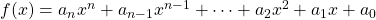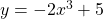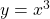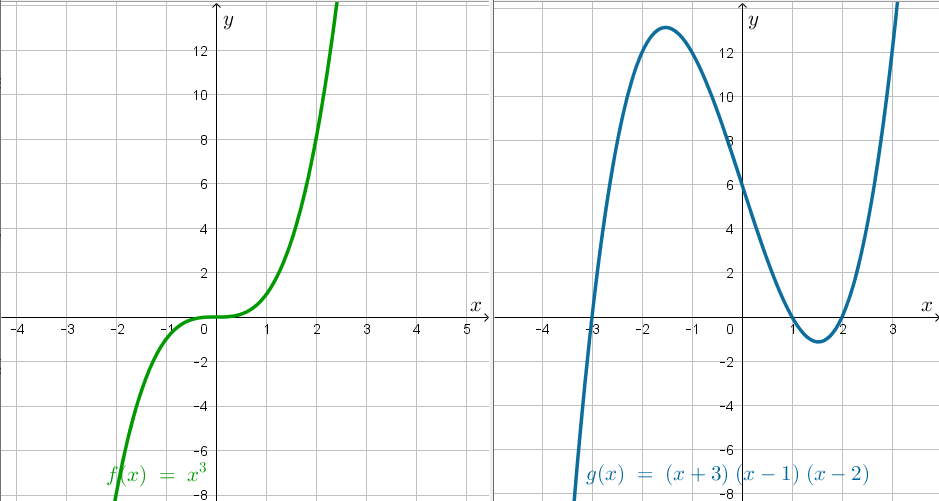# Polynomials Introduction

A polynomial of one variable is a function of the formwhere the coefficients of each term are real numbers and the exponents are positive integers. The graph of a polynomial function is a smooth and continuous curve, and can be drawn without raising pencil from paper.

# The family membersA constant (special case linear). Degree 0A linear function. Degree 1A quadratic function. Degree 2A cubic function. Degree 3A quartic function. Degree 4

…and so on. For polynomials of higher degree, we can look up the name or just refer to them by their degree, eg,is a ‘polynomial degree 5’.

The terms of a polynomial may be presented in any order, however the degree of the polynomial is defined as the largest exponent of.

# How many terms?

A polynomial of degree four will have between one and five terms. Examples include:The first function has just one term and is sometimes referred to as a monomial or a power function.

In general, a polynomial of degree four can be written asAs long as the coefficientis not zero, any combination of these terms is considered a polynomial of degree 4.

Using letters in order like this has its limitations, so an alternative presentation of a quartic is# Properties of the graph of a polynomial functionThe polynomial graphed above has the following features:

 Degree 5 (odd) End Behaviour Quartile 3 to Quartile 1 Zeros (roots) Three, at points A, B, C Turning Points Four, at points D and F (local max); E and G (local min). Constant term -11 (that is,-intercept)

The leading coefficient is the coefficient of the term with the highest power of. The sign of the leading coefficient () along with the degree of the polynomial (odd or even) determine the end behaviour of the graph of the polynomial.

# End Behaviour

Travelling from left to right, we say a graph ‘begins’ in one quadrant and ‘ends’ in another.

# Number of Zeros

Here is an example of a polynomial of degree 3. It is given in factored form:Finding the zeros from factored form is quite straightforward:A polynomial of degree 3 has at most 3 zeros.

Use the slider below to see how a cubic may have 3, 2 or 1 distinctintercepts.

In a similar way, we can determine the maximum and minimum number of zeros for polynomials of other degrees. We can createlinear factors to generate a polynomial of degreewithzeros which is the maximum number of zeros. The minimum number will either beor. It matters if the polynomial is an odd or an even degree polynomial.

# Number of Turning Points

A turning point on a graph is a point where the graph turns from rising to falling, from increasing to decreasing (or vice versa). The cubichas no turning points. The cubichas two turning points.As the maximum number of zeros (or intersections on any horizontal line) for a cubic is three, the maximum number of turning points is 2. Again, the minimum and maximum number of turning points is related to the degree, and again it matters if the polynomial is of odd or even degree.

# Investigate

Overlay the four arrow tool on an axis (to become ‘two arrows’), click and drag to change the scale of that axis.

Use the applet to create the following (if possible):

1. A quadratic with 2 zeros; a quadratic with 1 zero; a quadratic with no zeros. What is the number of turning points on the graph of a quadratic function?
2. A cubic with 3 zeros; with 2 zeros; with 1 zeros; with no zeros. What is the max/min number of turning points of a cubic?
3. A quartic with 4 zeros; with 3 zeros; with 2 zeros; with 1 zero; with no zeros. What is the max/min number of turning points on a quartic?

Use the applet to answer the following questions:

1. What generalisations can be made regarding zeros in polynomial degreecan be made?
2. What generalisations can be made regarding turning points in polynomial degreecan be made?
3. What generalisations can be made regarding the end behaviour of polynomial degree(odd/even?) with leading coefficient positive/negative?